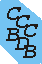Computational Chemistry Comparison and Benchmark DataBase Release 22 (May 2022) Standard Reference Database 101 National Institute of Standards and Technology Home All data for one species Geometry Experimental Calculated Comparisons Bad Calculations Tutorials and Explanations Vibrations Experimental Calculated Scale factors Reactions Entropies Ions List Ions Energy Electron Affinity Proton Affinity Ionization changes point group Experimental One molecule all properties One property a few molecules Geometry Vibrations Energy Electrostatics Reference Data Calculated Energy Optimized Reaction Internal Rotation Orbital Nuclear repulsion energy Correlation Ion Excited State Basis Set Extrapolation Geometry Vibrations Frequencies Zero point energy (ZPE) Scale Factors Bad Calculations Electrostatics Charges Dipole Quadrupole Polarizability Spin Entropy and Heat Capacity Reaction Lookup by property Comparisons Geometry Vibrations Energy Entropy Electrostatics Ion Resources Info on Results Calculations Done Basis functions used I/O files Glossary Conversion Forms Links NIST Links External links Thermochemistry Tutorials Vibrations Entropy Energy Electrostatics Geometry Cost Bad Calculations FAQ Help Units Choose Units Explanations Credits Just show me Summary Using List Recent molecules Molecules Geometry Vibrations Energy Similar molecules Ions, Dipoles, etc. Index of CCCBDB Feedback You are here: Calculated > Vibrations > Bad Calculations > Bad vib. Calc. vs exp. OR Resources > Bad Calculations > Vibrations > Bad vib. Calc. vs exp.

19 02 11 15 45

Calculated frequencies that differ from experiment by more than 400 cm-1 or have a ratio with experiment outside of 0.7 to 1.2 are listed

Calculated vibrational frequencies ("theory" in table) are calculated at M06-2X/CEP-31G
Calculated values were scaled by 1.

Species Name mode int rot Symmetry Experiment Theory difference ratio
SO2 Sulfur dioxide 1 A1 1151 952 -200 1.210
SO2 Sulfur dioxide 2 A1 518 410 -108 1.262
SO2 Sulfur dioxide 3 B2 1362 1046 -316 1.302
NH3 Ammonia 2 torsion A1 950 85 -865 11.186
NH3 Ammonia 3 E 3444 3920 476 0.879